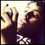# Superperfect Numbers form

$n$ is called a superperfect number when $\sigma(\sigma(n))=2n$.I saw every superperfect numbers is in the form $2^k$ when $2^{k+1}-1$ is a Mersenne prime.I can prove that numbers in this form will be superperfect but cannot prove the converse that all superperfect numbers are in this form. can anybody help me to prove? If this is not true then also tell me I am not sure about it.Note by Kalpok Guha
6 years, 4 months ago

This discussion board is a place to discuss our Daily Challenges and the math and science related to those challenges. Explanations are more than just a solution — they should explain the steps and thinking strategies that you used to obtain the solution. Comments should further the discussion of math and science.

When posting on Brilliant:

• Use the emojis to react to an explanation, whether you're congratulating a job well done , or just really confused .
• Ask specific questions about the challenge or the steps in somebody's explanation. Well-posed questions can add a lot to the discussion, but posting "I don't understand!" doesn't help anyone.
• Try to contribute something new to the discussion, whether it is an extension, generalization or other idea related to the challenge.

MarkdownAppears as
*italics* or _italics_ italics
**bold** or __bold__ bold
- bulleted- list
• bulleted
• list
1. numbered2. list
1. numbered
2. list
Note: you must add a full line of space before and after lists for them to show up correctly
paragraph 1paragraph 2

paragraph 1

paragraph 2

[example link](https://brilliant.org)example link
> This is a quote
This is a quote
    # I indented these lines
# 4 spaces, and now they show
# up as a code block.

print "hello world"
# I indented these lines
# 4 spaces, and now they show
# up as a code block.

print "hello world"
MathAppears as
Remember to wrap math in $$ ... $$ or $ ... $ to ensure proper formatting.
2 \times 3 $2 \times 3$
2^{34} $2^{34}$
a_{i-1} $a_{i-1}$
\frac{2}{3} $\frac{2}{3}$
\sqrt{2} $\sqrt{2}$
\sum_{i=1}^3 $\sum_{i=1}^3$
\sin \theta $\sin \theta$
\boxed{123} $\boxed{123}$

Sort by:

You mean you saw it on wiki? 'Cause its there...

- 6 years, 4 months ago

This might be helpful...check out perfect Numbers, as explained by Numberphile: (http://m.youtube.com/watch? v=q8n15q1v4Xo)

- 6 years, 4 months ago

Thank you very much it became really useful to me .Though here they only proved the form of perfect number but listening to the class I got idea to the form of superperfect number.Identified the series of super perfect number

- 6 years, 4 months ago

Can you please provide a proof that if $2^{k+1} -1$ is a Mersenne prime then $2^{k}$ is a perfect number because that might help us when we try to prove the converse.

- 6 years, 4 months ago

Here let $n=2^k$

$\sigma(\sigma(2^k)$

=$\sigma(\frac {2^{k+1}-1}{2-1})$

=$\sigma(2^{k+1}-1)$

As $2^{k+1}-1$ is a prime $\sigma(2^{k+1}-1)=(2^{k+1}-1)+1=2^{k+1}=2.2^k=2n$

Proved that it is always a superperfect number.

- 6 years, 4 months ago

Certainly I will post it .just wait

- 6 years, 4 months ago

I read it on Elementary Number Theory by David Burton,then opened wiki and saw but there is no proof there neither direct one nor converse

- 6 years, 4 months ago